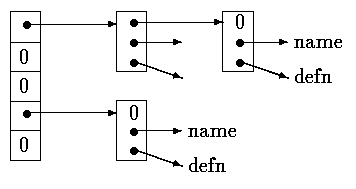# Programming Tutorials

## Table Lookup - hashtab - example program in C

By: Emiley J in C Tutorials on 2007-09-26

In this tutorial we will write the innards of a table-lookup package, to illustrate more aspects of structures. This code is typical of what might be found in the symbol table management routines of a macro processor or a compiler. For example, consider the #define statement. When a line like
```   #define  IN  1
```
is encountered, the name IN and the replacement text 1 are stored in a table. Later, when the name IN appears in a statement like
```   state = IN;
```
it must be replaced by 1.

There are two routines that manipulate the names and replacement texts. install(s,t) records the name s and the replacement text t in a table; s and t are just character strings. lookup(s) searches for s in the table, and returns a pointer to the place where it was found, or NULL if it wasn't there.

The algorithm is a hash-search - the incoming name is converted into a small non-negative integer, which is then used to index into an array of pointers. An array element points to the beginning of a linked list of blocks describing names that have that hash value. It is NULL if no names have hashed to that value.A block in the list is a structure containing pointers to the name, the replacement text, and the next block in the list. A null next-pointer marks the end of the list.

```   struct nlist {       /* table entry: */
struct nlist *next;   /* next entry in chain */
char *name;           /* defined name */
char *defn;           /* replacement text */
};
```
The pointer array is just
```   #define HASHSIZE 101

static struct nlist *hashtab[HASHSIZE];  /* pointer table */
```
The hashing function, which is used by both lookup and install, adds each character value in the string to a scrambled combination of the previous ones and returns the remainder modulo the array size. This is not the best possible hash function, but it is short and effective.
```   /* hash:  form hash value for string s */
unsigned hash(char *s)
{
unsigned hashval;

for (hashval = 0; *s != '\0'; s++)
hashval = *s + 31 * hashval;
return hashval % HASHSIZE;
}
```
Unsigned arithmetic ensures that the hash value is non-negative.

The hashing process produces a starting index in the array hashtab; if the string is to be found anywhere, it will be in the list of blocks beginning there. The search is performed by lookup. If lookup finds the entry already present, it returns a pointer to it; if not, it returns NULL.

```   /* lookup:  look for s in hashtab */
struct nlist *lookup(char *s)
{
struct nlist *np;

for (np = hashtab[hash(s)];  np != NULL; np = np->next)
if (strcmp(s, np->name) == 0)
return np;     /* found */
}
```
The for loop in lookup is the standard idiom for walking along a linked list:
```   for (ptr = head; ptr != NULL; ptr = ptr->next)
...
```
install uses lookup to determine whether the name being installed is already present; if so, the new definition will supersede the old one. Otherwise, a new entry is created. install returns NULL if for any reason there is no room for a new entry.
```   struct nlist *lookup(char *);
char *strdup(char *);

/* install:  put (name, defn) in hashtab */
struct nlist *install(char *name, char *defn)
{
struct nlist *np;
unsigned hashval;

np = (struct nlist *) malloc(sizeof(*np));
if (np == NULL || (np->name = strdup(name)) == NULL)
return NULL;
hashval = hash(name);
np->next = hashtab[hashval];
hashtab[hashval] = np;
} else       /* already there */
free((void *) np->defn);   /*free previous defn */
if ((np->defn = strdup(defn)) == NULL)
return NULL;
return np;
}
```